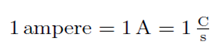## Pages

### Difference between Charge, Voltage & Currrent

Electrical Charge

Electrical charge is a fundamental quantity and is intrinsic property of matter which causes a force to act between particles (objects, bodies..) which have this property. It is physical quantity which can be transferred from one object to another.

Electric charge comes in two types, which wechoose to call positive charge and negative charge.

Electric charge can be measured using the law for the forces between charges (Coulomb’s Law). Charge is a scalar and is measured in coulombs. The coulomb is actually defined in terms of electric current (the flow of electrons), which is measured in amperes; when the current in a wire is 1 ampere, the amount of charge that flows past a given point in the wire in 1 second is 1 coulomb. Thus,when charges are transferred by simple interactions (i.e. rubbing), it is a negative charge which is transferred, and this charge is in the form of the fundamental particles called electrons. The charge of an electron(e) is -1.6022 × 10-19 C.

The particles found in nature all have charges which are integral multiples of the elementary charge e:
q = ne where n = 0, ±1, ±2 . . .. Because of this, we say that charge is quantized.

A charged object is an object that has an excess of one type of charge, e.g., more positive than negative. The amount of excess charge is the charge we assign to that object.

Current

Flow of charge moving through unit area in unit time. It represents charge transfer.

Voltage/Potential Difference

Voltage is a measure of the energy carried by the current. Technically it is a measure of the difference in energy between two points – hence the name potential difference. Voltage represents potential difference between two points. It represents work to be done to move unit charge from one point to other under influence of electric field.